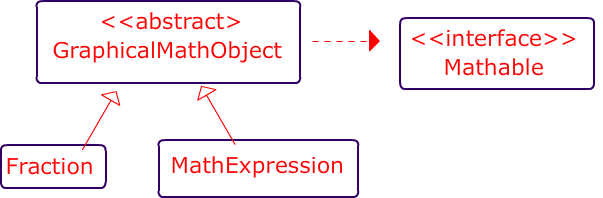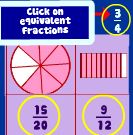# custom method go around lake

Objective: Make a jeroo walk around the perimeter of the lank, on the land side. Use a custom methoddownload the island file# Class Relationships involving interfaces and Abstract Classes

Two Caveats

1. all instance variables must be explicitly written as “private”
2. Declare ArrayList’s as type “list”:
1.  List<Integer> blahBlah = new ArrayList<Integer>()
2.The classes below represent different ways of representing mathematical  concepts like MathExpressions, Fractions etc. and they all implement the Mathable interface.### public abstract class GraphicalMathObject

methods

• public abstract void displayGraphicalRepresentation() ;

### public class Fraction extends GraphicalMathObject

This class is used to represent Fractions

Instance Variables

• private int numerator
• private int denominator

Constructor : The constructor should initialize both instance variables

• public Fraction(int num, int denom)

Methods

• public void displayGraphicalRepresentation() ; (just print out ” I’m a fraction -displayGraphicalRepresentation()”. This is the method that renders a graphical representation of the fraction .
•• private int getGCF() //returns the GCF of numerator and denominator
• public void simplify()//simplifies the fraction. Note: you must call getGCF()
• public Fraction clone() //return a clone of the current object (** Extra credit…research this) ( link )
• plus all of the methods in Mathable (Described at bottom)### public class MathExpression extends GraphicalMathObject

This is a class that is used to represent mathematical expressions like 3x2or 5x2y2 . It contains the methods and variables described below and it must implement Mathable

Instance Variables

• private int coefficient
• private List<String> variables
• private List<Integer> powers

NOTE: you should use the following sytnax

Declare ArrayList’s as type “list”:

1.  List<Integer> blahBlah = new ArrayList<Integer>()
2.Constructor : You can decide how to write the constructor.

• Just be sure to initialize the instance variables

methods implement all methods of the Mathable Interface in ways that make sense for this class. The idea with Mathable is that you should be able to tell if the objects are either equivalent, exactly the same and that you should be able to return a String representation (data) that uniquely identifies the given mathematical expression.

• public void addTerm( String term , int power) ) // This is used to represent multiplication, actually and this method adds a term with a power to the object’s list of `variables` and power to the list of `powers`. You can not assume that the term does not yet exist in the expression .
For instance, if the original term were 3x5 and the object called addTerm("y", 6)  the object would then be 3x5y6. Or for another example, if the original term is 2x3z, and  addTerm("z", 5)  the object would be 2x3z11 .
• public void displayGraphicalRepresentation()  ; (just print out “I”m a MathExpression.: displayGraphicalRepresentation()”). This is the method that draws the MathExpression on the screen like:
• plus all of the methods in Mathable

## Examples

 3x2y5 coefficient: 3 variables : [ “x”, “y”] powers : [ 2, 5] x1y11 coefficient: 1 variables : [ “x”, “y”] powers : [ 1, 11] 3y2x5 coefficient: 3 variables : [ “y”, “x”] powers : [ 5 , 2]### Interface : Mathable

• public String getData( ) ;   represents core mathematical information that defines current object.
• public boolean exactlyEquals(Mathable other) ;
• public boolean isEquivalent(Mathable other) ;

# Walk the Lake (custom Method)# The Betrothal

Tom and Tammy are in love, and today’s the day that Tom is going to propose. According to custom, Tom must present Tammy with a flower as an official sign of his intentions. Tom lives in the extreme northwest corner of the island, and Tammy lives in the extreme northeast corner. The dividing river runs north and south, dividing the island roughly in the middle; the river is at least 5 cells away from the western and eastern edges of the island. The river, itself, is exactly two cells wide. Fortunately, for the lovers, there is a bridge somewhere to the south of their homes. Tom has asked Tammy to meet him at the middle of the bridge. While she suspects his motives, she doesn’t want to appear too anxious. The purpose of this program is to have Tom and Tammy find the bridge and meet in the middle where Tom will give an engagement flower to Tammy. After he has given her the flower, each returns to its home and faces the home of its betrothed. Each Jeroo starts at its home, Tom at (0,0) and Tammy at (0,23). Each can start facing any direction. Tom starts with one special flower in his pouch. A representative starting layout is shown in figure 1Download the Island Files# Arrays II

• Arrays in Demo
• Test : June 10th/11th (Arrays and two dimensional arrays)
• Two Dimensional Arrays
• The problem with Arrays
• Array 2 (codingbat)  Do any 18 questions.  You can get extra credit for doing any of the last 4.  EOD Sunday  May 31st
• All students must do sum67
• Array Fun 2 (due by EOD Tuesday May 13rd )
• Array Fun 1 (email to me by EOD Tuesday May 6th)

# Arrays

• short circuiting
• Array 1 Coding Bat  type questions
• default values of arrays
• arrays in memory(value vs reference)
• swapping values in an array without creating a new array like this coding bat problem–assuming you didn’t make a new array
• Coding Bat : Array 1 (Thursday April 24th-that’s day of the 2nd class after the April break)
• Short Circuiting

April 2nd

• Test (April 2nd/3rd)
• All coding bat warm up and String 1’s must be completed by then
• Strings immutable, codingbat.com

# Strings (the basics)

## String Cheat Sheet``` String str ="ABCDEFG"```

` String firstLetter = str.substring(0,1)`

`String firstTwoLetters = str.substring(0,2)`

` String lastLttr = str.substring(str.length() -1)`

` String last_2_lttrs = str.substring(str.length() -2 )`

String middle_lttr = str.substring(str.length()/2 , str.length()/2 +1)
Unit 2

Extra credit: Looping over Strings

Unit I
Deadline the day you return from feb break

# Midterm Quiz

*Wed/Thursday 21st/22nd

• definitions:
• pass by value
• pass by reference
• C++ memory usage (see last asssessment) . Yes, pretty much same questions with different numbers
• floating point error
• understand of whether the following data types are pass by value vs reference in Java
• int
• double
• boolean